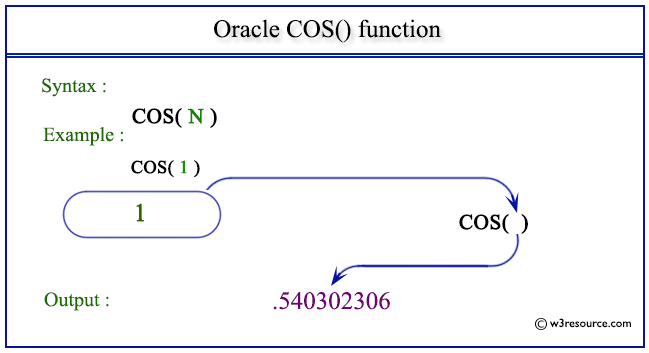# Oracle COS() function

## Description

The COS() function is used to get the cosine of a number where the number is given in radians.
The function takes any numeric or nonnumeric data type (can be implicitly converted to a numeric data type) as an argument.
If the argument is BINARY_FLOAT, then the function returns BINARY_DOUBLE. Otherwise, the function returns the same numeric data type as the argument

Syntax:

`COS(N)`

Parameters:

Name Description
N A number whose cosine value is to be retrieved.

Pictorial Presentation of COS() functionExample-1

The statement below will return the cosine of 180 degrees.

``````SELECT COS(180 * 3.14159265359/180) "Cosine 180 degrees"
FROM DUAL;
```
```

Here is the result.

```Cosine 180 degrees
------------------
-1
```

Example-2

The statement below will return the cosine of the number 1.

``````SELECT COS(1) "Cosine"
FROM DUAL;
```
```

Here is the result.

```    COS(1)
----------
.540302306
```

Previous: CEIL
Next: COSH

﻿